# Derive Ascent formula

Derive Ascent formula.

As the liquid rises, its weight increases and at a particular height say h , the force due to surface tension in the upward direction becomes equal to the weight of the liquid in the downward direction. The liquid then ceases to rise.
The weight of the liquid in the capillary, W = (Volume of cylindrical liquid column of height h + Volume of the liquid in meniscus) ? r ? g ,
where g is the acceleration due to gravity.
Now, volume of cylindrical liquid column = p r 2 h
For convenience, we consider the meniscus as to be hemispherical in shape.
Volume of the liquid in the meniscus = Volume of cylinder of height h and radius r - Volume of hemisphere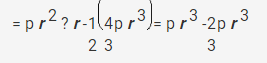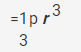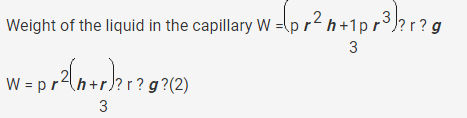In the equilibrium position, F = W
Equating equations (1) and (2), we get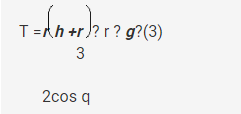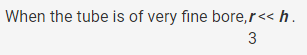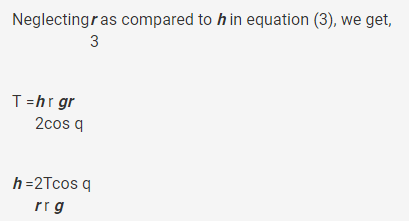This expression is called the ascent formula.